/

### Random Matrix Theory

Tools

References

The risks expressed by a portfolio of assets or liabilities depend heavily on how these assets and liabilities might ‘co-move’, i.e. move in tandem. One way of analysing these co-movement characteristics is to consider the covariances between the price movements of (or more precisely, the log returns on) different assets and liabilities.

Most usually, investigators encapsulate this information within a single structure, the covariance matrix, often estimating this matrix empirically, using past observed covariances between the different assets and liabilities.

But how do we tell how ‘reliable’ is this estimation process? Suppose we have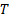time periods that we can use to estimate empirically the characteristics of the covariance matrix and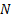assets or liabilities (i.e. ‘instruments’). The correlation matrix contains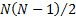distinct entries. Ifis large relative to(which it usually is in the risk management context) then we should expect the empirical determination of the covariance matrix to be ‘noisy’, i.e. for it to have a material element of randomness derived from measurement ‘noise’. It should therefore be used with caution. Such matrices can be characterised by their eigenvectors and eigenvalues, and it is the smallest (and hence apparently least relevant) of these that are most sensitive to this noise. But these are precisely the ones that determine, in Markowitz theory, the precise structure of optimal portfolios, see Laloux et al. (1999).

It is thus desirable to devise ways of distinguishing between ‘signal’ and ‘noise’, i.e. to distinguish between those eigenvalues and eigenvectors of the covariance matrix that appear to correspond to real characteristics exhibited by these assets and liabilities and those that are mere artefacts of this noise.

One way of doing this is to use random matrix theory. This theory has a long history in physics since Eugene Wigner and Freeman Dyson in the 1950s. It aims to characterise the statistical properties of the eigenvalues and eigenvectors of a given statistical ‘ensemble’ (i.e. the set of random matrices exhibiting pre-chosen symmetries or other sorts of constraints). Amongst other things, we might be interested in the average density of eigenvalues and in the distribution of spacing between consecutively ordered eigenvalues etc.

For example, we might compare the properties of an empirical covariance matrix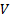with a ‘null hypothesis’ that the assets were, in fact, uncorrelated. Deviations from this null hypothesis that were sufficiently unlikely might then suggest the presence of true information.

In the limit of very large matrices (i.e.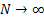) this density is analytically tractable and is as follows, for covariance matrices derived from (independent) series that have a common standard deviation,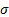. Correlation matrices derived from the above null hypothesis have this property, as they correspond to covariance matrices where each series has a standard deviation in isolation of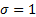.

The density, for a given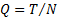, is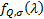where: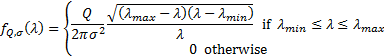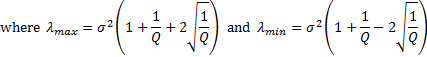In the limiting case whereis large this has some features that get smoothed out in practice for less extreme values of, in particular the existence of a hard upper and (if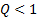) lower limit above and below which the density falls to zero.

We may therefore adopt the following prescription for ‘denoising’ an empirically observed correlation matrix, if we can assume that the ‘null’ hypothesis is that the instruments are independent (an assumption that would be inappropriate if, say, we should ‘expect’ two instruments to be correlated, e.g. the two might be listings of the same underlying asset on two different stock exchanges, or two bonds of similar terms issued by the same issuer), see Scherer (2007):

(a)    Work out the empirically observed correlation matrix (which by construction has standardised the return series so that).

(b)   Identify the largest eigenvalue and corresponding eigenvector of this matrix.

(c)    If the eigenvalue is sufficiently large, e.g. materially larger than the cut-off derived from the above (or some more accurate determination of the null hypothesis density applicable to the finitecase), then deem the eigenvalue to represent true information rather than noise and move on to step (d), otherwise stop.

(d)   Record the eigenvalue and corresponding eigenvector. Determine the contribution to each instrument’s return series from this eigenvector. Strip out these contributions from each individual instrument return series, calculate a new correlation matrix for these adjusted return series and loop back to (b).

The reason we in theory need to adjust each instrument return series in step (d) is that otherwise the ‘residual’ return series can no longer be assumed to have a common, so we can no longer directly use a formula akin to that above to identify further eigenvectors that appear to encapsulate true information rather than noise. However, if we ignore this nicety (i.e. we adopt the null hypothesis that all residual return series, after stripping out ‘significant eigenvectors’ are independent identically distributed Gaussian random series with equal standard deviations) then the computation of the cut-offs simplifies materially. This is because:

i.            The trace of a symmetric matrix (i.e. the sum of the leading diagonal elements) is the same as the sum of its eigenvectors (and is therefore invariant relative to a change in basis for the relevant vector space).

ii.            So, removing the leading eigenvector as above merely involves removing the leading row and column, if the basis used involves the eigenvectors.

iii.            The variance of the residual series under this null hypothesis is therefore merely the sum of the eigenvalues not yet eliminated iteratively.

iv.            Hence, we can calculate the cutoff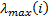for the’th eigenvalue (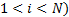as follows, where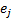is the magnitude of the’th eigenvalue (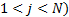: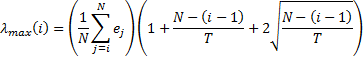Using this prescription, we would exclude any eigenvalues and corresponding eigenvectors beyond the first one for which the eigenvalue is not noticeably above this cutoff. The Nematrian website function MnEigenvalueSpreadsForRandomMatrices calculates these. More precise tests of significance could be identified by simulating spreads of results for random matrices.

Edelman and Rao (2005) describe how in many cases it is possible to calculate eigenvalue densities for a wide range of transformations of random matrices, including both deterministic and stochastic transformations. They express the view that the usefulness of random matrix theory will through time follow that of numerical analysis more generally, i.e. most disciplines in science and engineering will in due course find random matrix theory a valuable tool. Its history started in the physics of heavy atoms and multivariate statistics. It has already found its way into wireless communications and combinatorial mathematics and as seen above is potentially also becoming increasingly used in the field of financial analysis and risk management.